# PSEB 6th Class Maths Solutions Chapter 2 Whole Numbers Ex 2.3

Punjab State Board PSEB 6th Class Maths Book Solutions Chapter 2 Whole Numbers Ex 2.3 Textbook Exercise Questions and Answers.

## PSEB Solutions for Class 6 Maths Chapter 2 Whole Numbers Ex 2.3

1. If the product of two whole numbers is zero. Can we say that one or both of them will be zero? Justify through examples.
Solution:
One of them can be Zero i.e. 0 × 5 = 0
Both of them can be Zero i.e. 0 × 0 = 0.2. If the product of two whole numbers is 1. Can we say that one or both of them will be 1? Justify through examples.
Solution:
Both of them will be 1.
Example: 1 × 1 = 1.

3. Observe the pattern in the following and fill in the blanks: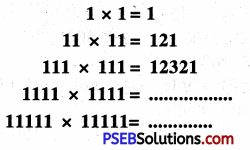Solution: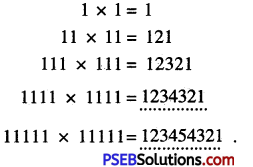4. Observe the pattern and fill in the blanks: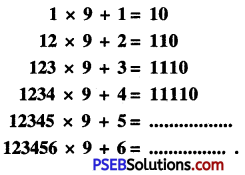Solution: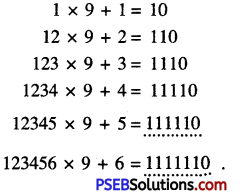5. Represent numbers from 24 to 30 according to rectangular, square or triangular pattern.
Solution:
Numbers from 24 to 30 are 24, 25, 26, 27, 28, 29, 30.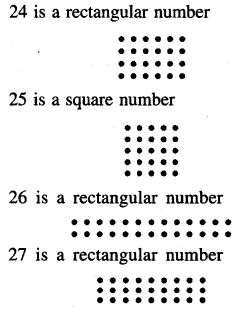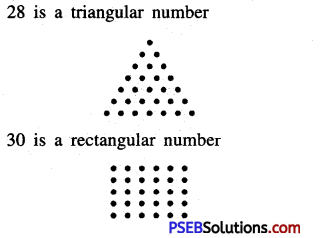6. Study the following pattern:

Question (i)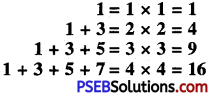Hence find the sum of
(a) First 12 odd numbers
(b) First 50 odd numbers.
Solution:
(a) Sum of first 12 odd numbers = 12 × 12 = 144
(b) Sum of first 50 odd numbers = 50 × 50 = 2500